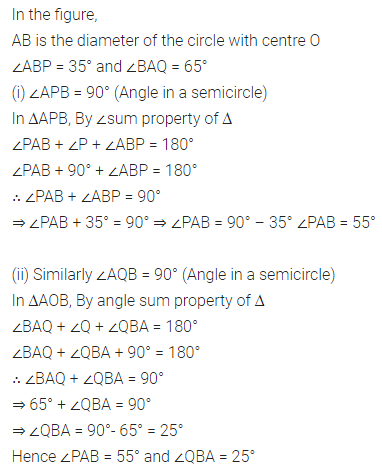## ML Aggarwal Class 8 Solutions for ICSE Maths Chapter 15 Circle Check Your Progress

Question 1.
Draw a circle of radius 2·7 cm. Draw a chord PQ of length 4 cm of this circle. Shade the major segment of this circle.
Solution:Question 2.
Draw a circle of radius 3·2 cm and in it make a sector of angle.
(i) 30°
(ii) 135°
(iii) $$2 \frac{2}{3}$$ right angles
Draw separate diagrams and shade the sectors.
Solution: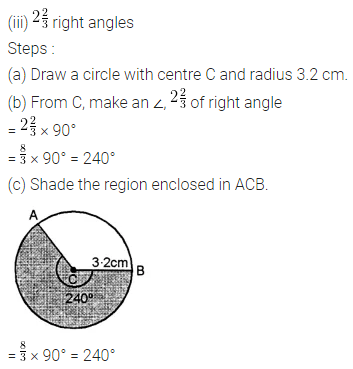Question 3.
Draw a line segment PQ = 6·4 cm. Construct a circle on PQ as diameter. Take any point R on this circle and measure ∠PRQ.
Solution: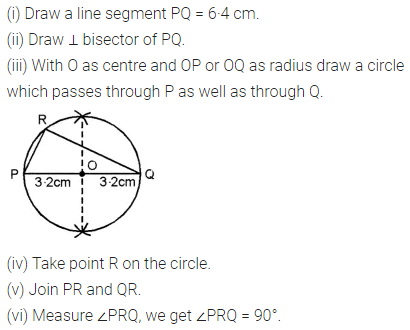Question 4.
In the adjoining figure, the tangent to a circle of radius 6 cm from an external point P is of length 8 cm. Calculate the distance of the point P from the nearest point of the circumference.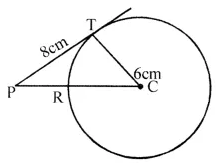Solution: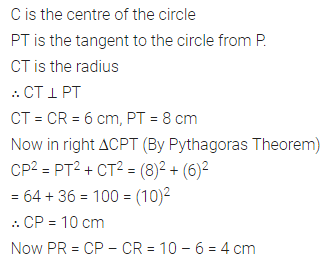Question 5.
In the given figure, O is the centre of the circle. If ∠ABP = 35° and ∠BAQ = 65°, find
(i) ∠PAB
(ii) ∠QBA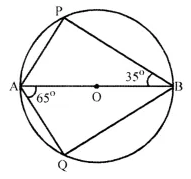Solution: